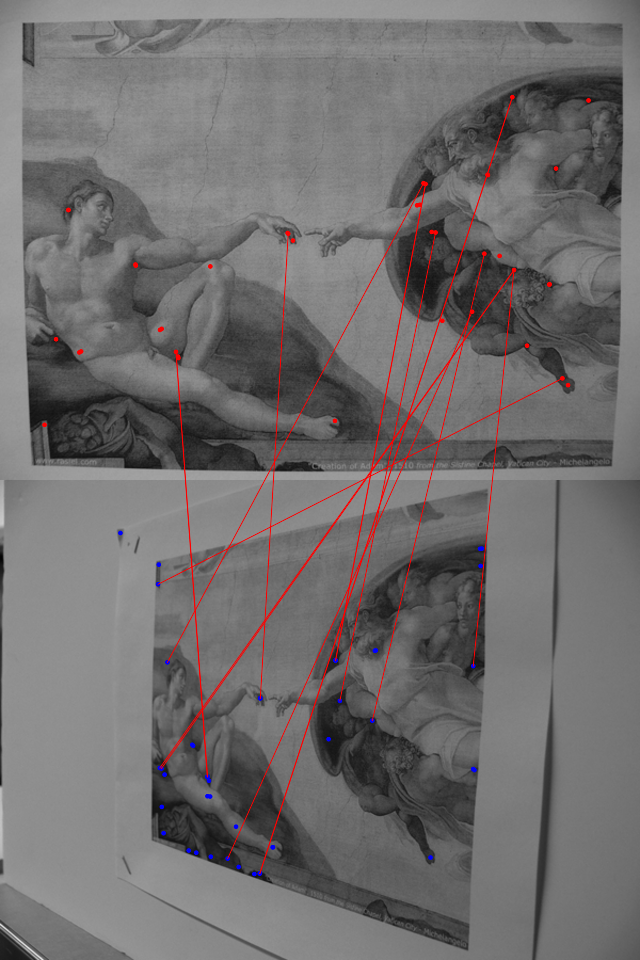# image.dlib

The image.dlib package allows to quickly obtain image features (SURF and HOG features) and to do image matching.

## Examples``````library(magick)
library(image.dlib)

height <- image_info(img1)\$height``````
``````## Get the surf descriptors
sp1 <- image_surf(image_data(img1, channels = "rgb"), max_points = 50)
sp2 <- image_surf(image_data(img2, channels = "rgb"), max_points = 50)

## Match surf points
library(FNN)
knn <- get.knnx(sp1\$surf, sp2\$surf, k = 1)

## Plot the 2 images and the top 15 matches of the SURF points
combined <- image_append(c(img1, img2), stack = TRUE)
plt <- image_draw(combined)
points(sp1\$x, sp1\$y, col = "red", pch = 20)
points(sp2\$x, sp2\$y + height, col = "blue", pch = 20)
• Look to the documentation of the R package for examples: `help(package = image.dlib)`